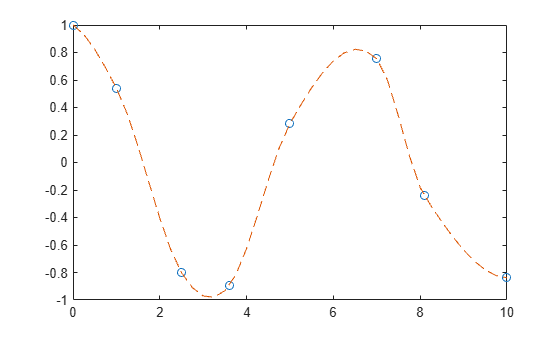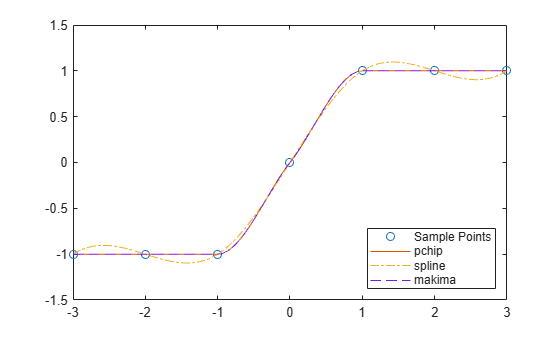# makima

## 语法

``yq = makima(x,y,xq)``
``pp = makima(x,y)``

## 说明

``yq = makima(x,y,xq)` 使用采样点 `x` 处的值 `y` 执行修正 Akima 插值，以求出查询点 `xq` 处的插值 `yq`。`

``pp = makima(x,y)` 返回一个分段多项式结构体以用于 `ppval` 和样条实用工具 `unmkpp`。`

## 示例

```x = [0 1 2.5 3.6 5 7 8.1 10]; y = cos(x); xq = 0:.25:10; yq = makima(x,y,xq); plot(x,y,'o',xq,yq,'--')```Akima 算法利用振荡函数使局部极值附近的曲线平坦化。为了补偿这种平坦化，您可以在局部极值附近添加更多采样点。

$\mathit{x}=6.5$$\mathit{x}=9$ 附近添加采样点，然后重新绘制插值。

```x = [0 1 2.5 3.6 5 6.5 7 8.1 9 10]; y = cos(x); xq = 0:.25:10; yq = makima(x,y,xq); plot(x,y,'o',xq,yq,'--')````spline``pchip``makima` 为两个不同数据集生成的插值结果进行比较。这些函数都执行不同形式的分段三次 Hermite 插值。每个函数计算插值斜率的方式不同，因此它们在基础数据的平台区或波动处展现出不同行为。

```x = -3:3; y = [-1 -1 -1 0 1 1 1]; xq1 = -3:.01:3; p = pchip(x,y,xq1); s = spline(x,y,xq1); m = makima(x,y,xq1); plot(x,y,'o',xq1,p,'-',xq1,s,'-.',xq1,m,'--') legend('Sample Points','pchip','spline','makima','Location','SouthEast')``````x = 0:15; y = besselj(1,x); xq2 = 0:0.01:15; p = pchip(x,y,xq2); s = spline(x,y,xq2); m = makima(x,y,xq2); plot(x,y,'o',xq2,p,'-',xq2,s,'-.',xq2,m,'--') legend('Sample Points','pchip','spline','makima')``````x = -5:5; y = [1 1 1 0 0 1 1 2 2 2 2]; pp = makima(x,y)```
```pp = struct with fields: form: 'pp' breaks: [-5 -4 -3 -2 -1 0 1 2 3 4 5] coefs: [10x4 double] pieces: 10 order: 4 dim: 1 ```

```xq = -5:0.2:5; m = ppval(pp,xq); plot(x,y,'o',xq,m,'-.') ylim([-0.2 2.2])```## 输出参数

• 如果 `y` 为向量，则 `yq` 的大小与 `xq` 相同。

• 如果 `y` 是大小为 `Ny = size(y)` 的数组，则下列条件适用：

• 如果 `xq` 为标量或向量，则 `size(yq)` 返回 `[Ny(1:end-1) length(xq)]`

• 如果 `xq` 是数组，则 `size(yq)` 返回 `[Ny(1:end-1) size(xq)]`

`form`

`'pp'`分段多项式

`breaks`

`coefs`

`L`×`k` 矩阵，其中每行 `coefs(i,:)` 包含第 `i` 个区间 `[breaks(i),breaks(i+1)]``k` 次多项式的局部系数

`pieces`

`order`

`dim`

`$f\left(x\right)=a{\left(x-{x}_{1}\right)}^{3}+b{\left(x-{x}_{1}\right)}^{2}+c\left(x-{x}_{1}\right)+d\text{\hspace{0.17em}}.$`

## 详细信息

### 修正 Akima 插值

 中所述的一维插值 Akima 算法执行三次插值以生成具有连续一阶导数 (C1) 的分段多项式。该算法可避免局部过度波动。

`${d}_{i}=\frac{{w}_{1}}{{w}_{1}+{w}_{2}}{\delta }_{i-1}+\frac{{w}_{2}}{{w}_{1}+{w}_{2}}{\delta }_{i}.$`

`$\begin{array}{l}{w}_{1}=|{\delta }_{i+1}-{\delta }_{i}|,\\ {w}_{2}=|{\delta }_{i-1}-{\delta }_{i-2}|.\end{array}$`

`$\begin{array}{l}{w}_{1}=|{\delta }_{i+1}-{\delta }_{i}|+\frac{|{\delta }_{i+1}+{\delta }_{i}|}{2},\\ {w}_{2}=|{\delta }_{i-1}-{\delta }_{i-2}|+\frac{|{\delta }_{i-1}+{\delta }_{i-2}|}{2}.\end{array}$`

`spline` 算法相比，Akima 算法产生的波动较少，更适合处理平台区之间的快速变化。与 `pchip` 算法相比，Akima 算法不那么急剧平坦化，因此仍然能够处理振荡数据。

 Akima, Hiroshi. "A new method of interpolation and smooth curve fitting based on local procedures." Journal of the ACM (JACM) , 17.4, 1970, pp. 589–602.

 Akima, Hiroshi. "A method of bivariate interpolation and smooth surface fitting based on local procedures." Communications of the ACM , 17.1, 1974, pp. 18–20.